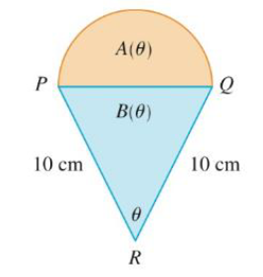Chapter 3.3, Problem 56E### Single Variable Calculus: Early Tr...

8th Edition
James Stewart
ISBN: 9781305270343

#### Solutions

Chapter
Section### Single Variable Calculus: Early Tr...

8th Edition
James Stewart
ISBN: 9781305270343
Textbook Problem

# A semicircle with diameter PQ sits on an isosceles triangle PQR to form a region shaped like a two-dimensional ice-cream cone, as shown in the figure. If A(θ) is the area of the semicircle and B(θ) is the area of the triangle, find. lim θ → 0 +   A ( θ ) B ( θ )To determine

To find: The value of limθ0+A(θ)B(θ) .

Explanation

Given:

The area of the semicircle is A(θ) and the area of the triangle is B(θ).

Formula used:

The area of the semicircle is 12πr2 (1)

The area of the triangle is 12bh (2)

Calculation:

The given picture is shown in the below Figure 1.

From Figure 1, the base of the triangle (PQ) is same as the diameter of semicircle (2r).

Calculate r and h.

From the triangle PSR,

sinθ2=opposite sidehypotenuse=r10r=10sinθ2

And

Substitute b=2r in equation (2),

B(θ)=12(2r)h=rh

Thus, the area of the triangle is B(θ)=rh.

From equation (1), the area if the semicircle is A(θ)=12πr2.

Consider limθ0+A(θ)B(θ)

### Still sussing out bartleby?

Check out a sample textbook solution.

See a sample solution

#### The Solution to Your Study Problems

Bartleby provides explanations to thousands of textbook problems written by our experts, many with advanced degrees!

Get Started

#### Solve the equations in Exercises 126. 14x2=0

Finite Mathematics and Applied Calculus (MindTap Course List)

#### Given that the point P(2, 3) lies on the line 2x + ky + 10 = 0, find k.

Applied Calculus for the Managerial, Life, and Social Sciences: A Brief Approach

#### In problems 9-18, simplify the expressions with all exponents positive. 13.

Mathematical Applications for the Management, Life, and Social Sciences